Documentation

## R Wave Detection in the ECG

This example shows how to use wavelets to analyze electrocardiogram (ECG) signals. ECG signals are frequently nonstationary meaning that their frequency content changes over time. These changes are the events of interest.

Wavelets decompose signals into time-varying frequency (scale) components. Because signal features are often localized in time and frequency, analysis and estimation are easier when working with sparser (reduced) representations.

The QRS complex consists of three deflections in the ECG waveform. The QRS complex reflects the depolarization of the right and left ventricles and is the most prominent feature of the human ECG.

Load and plot an ECG waveform where the R peaks of the QRS complex have been annotated by two or more cardiologists. The ECG data and annotations are taken from the MIT-BIH Arrhythmia Database. The data are sampled at 360 Hz.

```load mit200 figure plot(tm,ecgsig) hold on plot(tm(ann),ecgsig(ann),'ro') xlabel('Seconds') ylabel('Amplitude') title('Subject - MIT-BIH 200')```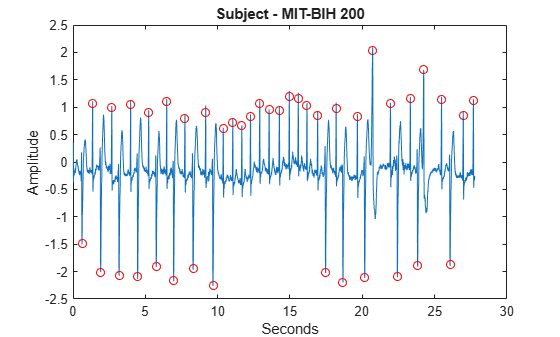You can use wavelets to build an automatic QRS detector for use in applications like R-R interval estimation.

There are two keys for using wavelets as general feature detectors:

• The wavelet transform separates signal components into different frequency bands enabling a sparser representation of the signal.

• You can often find a wavelet which resembles the feature you are trying to detect.

The 'sym4' wavelet resembles the QRS complex, which makes it a good choice for QRS detection. To illustrate this more clearly, extract a QRS complex and plot the result with a dilated and translated 'sym4' wavelet for comparison.

```qrsEx = ecgsig(4560:4810); [mpdict,~,~,longs] = wmpdictionary(numel(qrsEx),'lstcpt',{{'sym4',3}}); figure plot(qrsEx) hold on plot(2*circshift(mpdict(:,11),[-2 0]),'r') axis tight legend('QRS Complex','Sym4 Wavelet') title('Comparison of Sym4 Wavelet and QRS Complex')```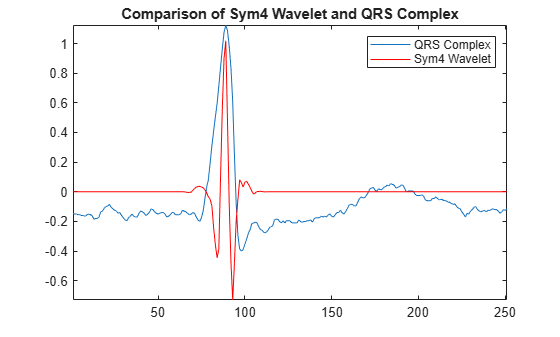Use the maximal overlap discrete wavelet transform (MODWT) to enhance the R peaks in the ECG waveform. The MODWT is an undecimated wavelet transform, which handles arbitrary sample sizes.

First, decompose the ECG waveform down to level 5 using the default 'sym4' wavelet. Then, reconstruct a frequency-localized version of the ECG waveform using only the wavelet coefficients at scales 4 and 5. The scales correspond to the following approximate frequency bands.

• Scale 4 -- [11.25, 22.5) Hz

• Scale 5 -- [5.625, 11.25) Hz.

This covers the passband shown to maximize QRS energy.

```wt = modwt(ecgsig,5); wtrec = zeros(size(wt)); wtrec(4:5,:) = wt(4:5,:); y = imodwt(wtrec,'sym4');```

Use the squared absolute values of the signal approximation built from the wavelet coefficients and employ a peak finding algorithm to identify the R peaks.

If you have the Signal Processing Toolbox™, you can use `findpeaks` to locate the peaks. Plot the R-peak waveform obtained with the wavelet transform annotated with the automatically-detected peak locations.

```y = abs(y).^2; [qrspeaks,locs] = findpeaks(y,tm,'MinPeakHeight',0.35,... 'MinPeakDistance',0.150); figure plot(tm,y) hold on plot(locs,qrspeaks,'ro') xlabel('Seconds') title('R Peaks Localized by Wavelet Transform with Automatic Annotations')```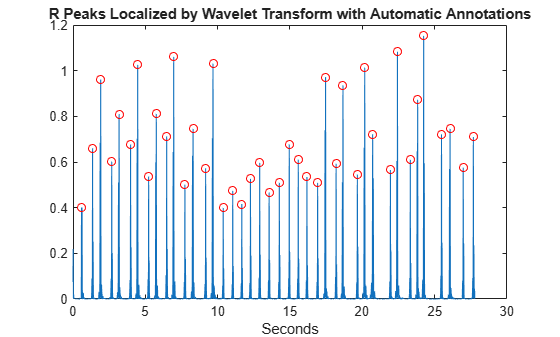Add the expert annotations to the R-peak waveform. Automatic peak detection times are considered accurate if within 150 msec of the true peak ($±75$ msec).

```plot(tm(ann),y(ann),'k*') title('R peaks Localized by Wavelet Transform with Expert Annotations')```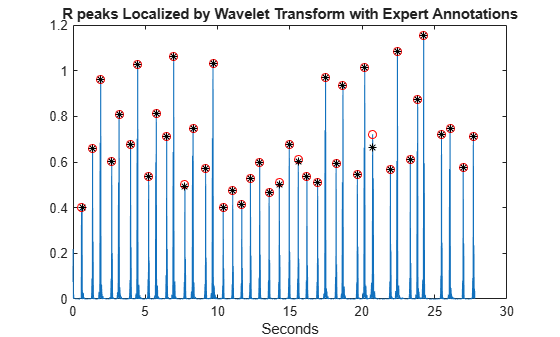At the command line, you can compare the values of `tm(ann)` and `locs`, which are the expert times and automatic peak detection times respectively. Enhancing the R peaks with the wavelet transform results in a hit rate of 100% and no false positives. The calculated heart rate using the wavelet transform is 88.60 beats/minute compared to 88.72 beats/minute for the annotated waveform.

If you try to work on the square magnitudes of the original data, you find the capability of the wavelet transform to isolate the R peaks makes the detection problem much easier. Working on the raw data can cause misidentifications such as when the squared S-wave peak exceeds the R-wave peak around 10.4 seconds.

```figure plot(tm,ecgsig,'k--') hold on plot(tm,y,'r','linewidth',1.5) plot(tm,abs(ecgsig).^2,'b') plot(tm(ann),ecgsig(ann),'ro','markerfacecolor',[1 0 0]) set(gca,'xlim',[10.2 12]) legend('Raw Data','Wavelet Reconstruction','Raw Data Squared', ... 'Location','SouthEast'); xlabel('Seconds')```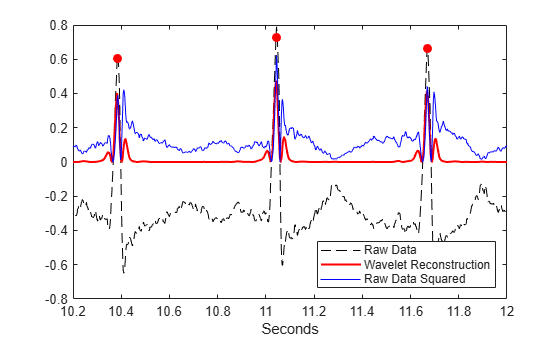Using `findpeaks` on the squared magnitudes of the raw data results in twelve false positives.

```[qrspeaks,locs] = findpeaks(ecgsig.^2,tm,'MinPeakHeight',0.35,... 'MinPeakDistance',0.150);```

In addition to switches in polarity of the R peaks, the ECG is often corrupted by noise.

```load mit203 figure plot(tm,ecgsig) hold on plot(tm(ann),ecgsig(ann),'ro') xlabel('Seconds') ylabel('Amplitude') title('Subject - MIT-BIH 203 with Expert Annotations')```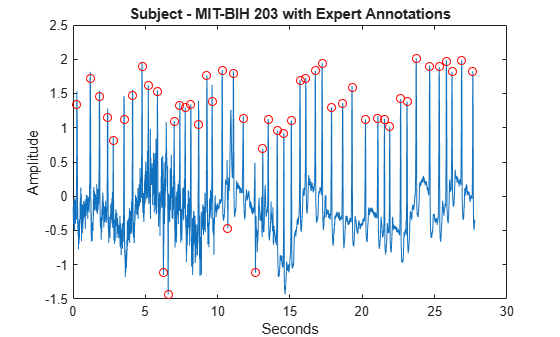Use the MODWT to isolate the R peaks. Use `findpeaks` to determine the peak locations. Plot the R-peak waveform along with the expert and automatic annotations.

```wt = modwt(ecgsig,5); wtrec = zeros(size(wt)); wtrec(4:5,:) = wt(4:5,:); y = imodwt(wtrec,'sym4'); y = abs(y).^2; [qrspeaks,locs] = findpeaks(y,tm,'MinPeakHeight',0.1,... 'MinPeakDistance',0.150); figure plot(tm,y) title('R-Waves Localized by Wavelet Transform') hold on hwav = plot(locs,qrspeaks,'ro'); hexp = plot(tm(ann),y(ann),'k*'); xlabel('Seconds') legend([hwav hexp],'Automatic','Expert','Location','NorthEast');```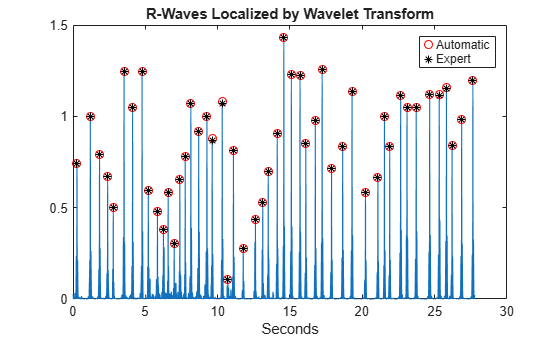The hit rate is again 100% with zero false alarms.

The previous examples used a very simple wavelet QRS detector based on a signal approximation constructed from modwt. The goal was to demonstrate the ability of the wavelet transform to isolate signal components, not to build the most robust wavelet-transform-based QRS detector. It is possible, for example, to exploit the fact that the wavelet transform provides a multiscale analysis of the signal to enhance peak detection.

References

Goldberger A. L., L. A. N. Amaral, L. Glass, J. M. Hausdorff, P. Ch. Ivanov, R. G. Mark, J. E. Mietus, G. B. Moody, C-K Peng, H. E. Stanley. "PhysioBank, PhysioToolkit, and PhysioNet: Components of a New Research Resource for Complex Physiologic Signals." Circulation 101. Vol.23, e215-e220, 2000. `http://circ.ahajournals.org/cgi/content/full/101/23/e215`

Moody, G. B. "Evaluating ECG Analyzers". `http://www.physionet.org/physiotools/wfdb/doc/wag-src/eval0.tex`

Moody G. B., R. G. Mark. "The impact of the MIT-BIH Arrhythmia Database." IEEE Eng in Med and Biol. Vol. 20, Number 3, 2001), pp. 45-50 .

##### Support평가판 신청